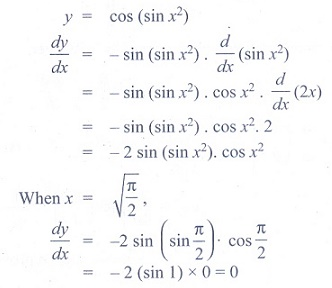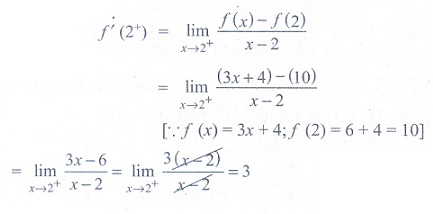Home | | Maths 11th std | Exercise 10.5: Choose the correct answer

# Exercise 10.5: Choose the correct answer

Maths Book back answers and solution for Exercise questions - Mathematics : Differential Calculus- Differentiability and Methods of Differentiation

Differentiability and Methods of Differentiation - Differential Calculus (Mathematics)

Choose the correct or the most suitable answer from the given four alternatives.

(1)  d/ dx (2/π sin x° ) oris

(1) π/180 . cos x°

(2) 1/90 . cos x°

(3) π/90 . cos x°

(4) 2/ π . cos x°

Ans: (2)

Solution(2) If y = f (x2 + 2) and f '(3) = 5, then dy/dx at x = 1 is

(1) 5

(2) 25

(3) 15

(4) 10

Ans: (4)

Solution(3) If y = 1/4  u4 , u = 2/3  x3 + 5, then dy/dx is

(1) 1/ 27 x2 (2x3 +15)3

(2) 2/27 x (2x3 + 5)3

(3) 2/ 27  x2 (2x3 +15)3

(4) 2/27  x (2x3 + 5)3

Ans: (3)

Solution(4) If f (x) = x2 − 3x , then the points at which f (x) = f '(x) are

(1) both positive integers

(2) both negative integers

(3) both irrational

(4) one rational and another irrational

Ans: (3)

Solution(5) If y = 1/ a − z , then dz/ dy is

(1) (a − z)2

(2) −( z − a)2

(3) (z + a)2

(4) − ( z + a)2

Ans: (1)

Solution(6) If y = cos(sin x2), then dy/dx at x = √[π/2] is

(1) - 2

(2) 2

(3) −2 √[π/2]

(4) 0

Ans: (4)

Solution(7) If y = mx + c and f (0) = f '(0) = 1, then f (2) is

(1) 1

(2) 2

(3) 3

(4) - 3

Ans: (3)

Solution(8) If f (x) = x tan−1x , then f '(1) is

(1) 1+ π/4

(2) 1/2 + π/4

(3) 1/2 – π/4

(4) 2

Ans: (2)

Solution(9) d/ dx (ex +5logx) is

(1) ex .x4 (x + 5)

(2) ex .x (x + 5)

(3) ex + 5/x

(4) ex -  5/x

Ans: (1)

Solution(10) If the derivative of (ax − 5)e3x at x = 0 is -13, then the value of a is

(1) 8

(2) - 2

(3) 5

(4) 2

Ans: (4)

Solution(11)(1) −y /x

(2) y/x

(3) – x/y

(4) x/y

Ans: (3)

Solution(12) If x = asinθ and y = bcosθ , then d2y/ d2x is

(1) a/ b2 sec2θ

(2) – b/a sec2θ

(3) –b/a2 sec3θ

(4) −b2/a2  sec3θ

Ans: (3)

Solution(13) The differential coefficient of log10x with respect to logx10 is

(1) 1

(2) −(log10x)2

(3) (logx10)2

(4) x2 /100

Ans: (2)

Solution(14) If f (x) = x + 2, then f '( f (x)) at x = 4 is

(1) 8

(2) 1

(3) 4

(4) 5

Ans: (2)

Solution(15) If y = (1− x)2/ x2 , then dy/ dx is

(1) 2/x2 + 2/x3

(2) −2/x2 + 2/x3

(3) −2/x2 − 2/x3

(4) −2/x2 + 2/x3

Ans: (4)

Solution(16) If pv = 81, then dp/dv at v = 9 is

(1) 1

(2) - 1

(3) 2

(4) -2

Ans: (2)

Solution(17) If  f (x ) =, then the right hand derivative of f(x) at x = 2 is

(1) 0

(2) 2

(3) 3

(4) 4

Ans: (3)

Solution(18) It is given that f '(a) exists, then lim x →a [xf (a ) − af (x)]/[ x − a] is

(1) f (a ) − af '(a)

(2) f '(a)

(3) − f '(a)

(4) f (a ) + af '(a)

Ans: (1)

Solution(19) If, then f '(2) is

(1) 0

(2) 1

(3) 2

(4) does not exist

Ans: (4)

Solution(20) If g (x) = (x2 + 2x + 3) f (x) and f (0) = 5 and, then g '(0) is

(1) 20

(2) 14

(3) 18

(4) 12

Ans: (2)

(21) If, then at x = 3, f '(x) is

(1) 1

(2) - 1

(3) 0

(4) does not exist

Ans: (4)

Solution(22) The derivative of f (x) = x | x | at x = −3 is

(1) 6

(2) - 6

(3) does not exist

(4) 0

Ans: (1)

Solution(23) If f (x), then which one of the following is true?

(1) f(x) is not differentiable at x = a

(2) f(x) is discontinuous at x = a

(3) f(x) is continuous for all x in R

(4) f(x) is differentiable for all xa

Ans: (1)

Solution(24) If f (x)is differentiable at x = 1, then

(1) a = 1/2 , b = −3/2

(2) a = −1/2 , b = 3/2

(3) a = − 1/2 , b = − 3/2

(4) a = 1/2 , b = 3/2

Ans: (3)

Solution(25) The number of points in R in which the function f (x) =| x − 1| + | x − 3| + sin x is not differentiable, is

(1) 3

(2) 2

(3) 1

(4) 4

Ans: (2)

SolutionTags : Differentiability and Methods of Differentiation | Differential Calculus | Mathematics , 11th Mathematics : UNIT 10 : Differential Calculus: Differentiability and Methods of Differentiation
Study Material, Lecturing Notes, Assignment, Reference, Wiki description explanation, brief detail
11th Mathematics : UNIT 10 : Differential Calculus: Differentiability and Methods of Differentiation : Exercise 10.5: Choose the correct answer | Differentiability and Methods of Differentiation | Differential Calculus | Mathematics